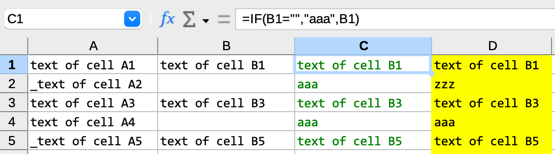# Formula with combined IF functions

I am working on a large spreadsheet and need a formula to be used in all the cells of a particular column.

Let’s say there are 4 columns; columns A and B consist of plain text cells, while column C consists of cells with a formula. What I need is the correct formula to use in column D:sampleIF.ods (9.7 KB)

• the cells of column A consist of a text string that may start with an underscore (_)
• the cells of column B are blank or consist of a text string
• the cells of column C contain the text string `aaa` if cell B on the same row is blank; otherwise, they contain the same text string as cell B on the same row
• the cells of column D contain the text string `zzz` if cell A on the same row starts with an underscore (_) and if cell C on the same row is `aaa`; otherwise, they contain the same text string as cell C on the same row

Any help would be greatly appreciated!

perhaps edit your question with what you have tried so far and the result - even better, share a sample sheet with the requirement.

1 Like

It looks like this should work for you.

`=IF(AND(LEFT(A1,1)="_",C1="aaa"),"zzz",C1)`

2 Likes

This works as expected. Thank you so much!